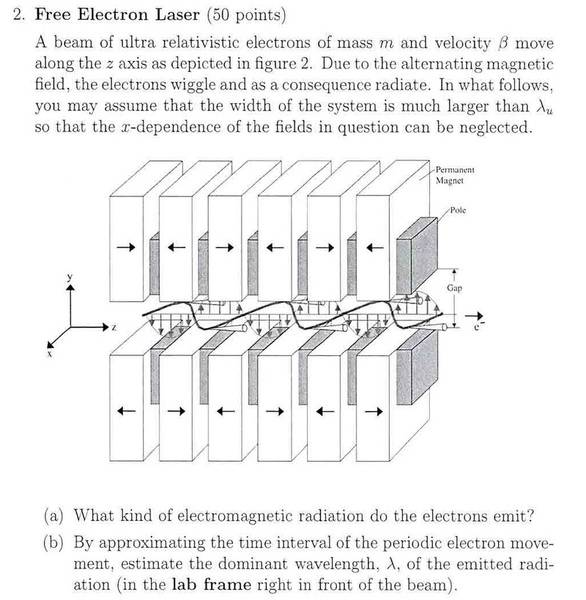# Free Electron Laser

Raz91

## Homework Statement## Homework Equations

2. (b)

I didn't understand how can I find the app. wavelength

## The Attempt at a Solution

I tried : the electron move over λu with velocity β so the wavelength is λ≈λu
but its not the right answer.

my lecturer told me I should consider also the dopler

I only know that the answer is :
λ≈λu /(2*γ2)

γ= gamma
THANK YOU!

## Answers and Replies

Mentor
I tried : the electron move over λu with velocity β so the wavelength is λ≈λu
This is the distance the light can travel while the electrons move by 1 structure length - but then the electrons moved forwards as well. What is the difference between those two distances?

Raz91
the electron moves λu while the light moves λ≈λu /β
so the difference is λu [1/β-1]

so how can I use this fact to find the wave length?

I tried use the approximation β≈1-1q/(2*γ^2) for ultra-relativistic electrons
but still didnt get the right answer....

thank you

Mentor
1/β is also 1/(1+β-1) where β-1 is small, so you can express that fraction as ~1-β+1
That should lead to the right answer.